# A Quick Introduction to iNEXT.3D via Examples

#### 2023-09-06

## install iNEXT.3D package from CRAN
# install.packages("iNEXT.3D")  # coming soon

install.packages('devtools')
library(devtools)
install_github('AnneChao/iNEXT.3D')

## import packages
library(iNEXT.3D)
library(ggplot2)

In this document, here provide a quick introduction demonstrating how to run the package iNEXT.3D (iNterpolation and EXTrapolation in three Dimensions). iNEXT.3D has several main functions: iNEXT3D, ggiNEXT3D, AO3D, ggAO3D, estimate3D, and DataInfo3D.

iNEXT.3D is the extension of R package iNEXT (Hsieh et al., 2016). iNEXT.3D focuses on three measures of Hill numbers of order q: species richness (q = 0), Shannon diversity (q = 1, the exponential of Shannon entropy) and Simpson diversity (q = 2, the inverse of Simpson concentration) and extend Hill number to three dimensions: taxonomic diversity (TD), phylogenetic diversity (PD), and functional diversity (FD) under Hill-Chao family frame work (Chao et al., 2019). Besides, iNEXT.3D also provide statistic estimation for three dimensions biodiversity (Chao et al., 2021). For each diversity measure, iNEXT.3D uses the observed sample of abundance or incidence data (called the “reference sample”) to compute diversity estimates and the associated confidence intervals for the following two types of rarefaction and extrapolation (R/E):

1. Sample-size-based R/E sampling curves:iNEXT3D computes diversity estimates for rarefied and extrapolated samples up to an appropriate size. This type of sampling curve plots the diversity estimates with respect to sample size.
2. Coverage-based R/E sampling curves: iNEXT3D computes diversity estimates for rarefied and extrapolated samples with sample completeness (as measured by sample coverage) up to an appropriate coverage. This type of sampling curve plots the diversity estimates with respect to sample coverage.

iNEXT.3D also plots the above two types of sampling curves and a sample completeness curve by ggiNEXT3D. The sample completeness curve provides a bridge between these two types of curves.

## How to cite

If you publish your work based on results from iNEXT.3D package, you should make references to the following Online reference:

• Chao, A., Henderson, P. A., Chiu, C.-H., Moyes, F., Hu, K.-H., Dornelas, M and. Magurran, A. E. (2021). Measuring temporal change in alpha diversity: a framework integrating taxonomic, phylogenetic and functional diversity and the iNEXT.3D standardization. Methods in Ecology and Evolution, 12, 1926-1940.

## HOW TO RUN iNEXT.3D:

The iNEXT.3D package can be downloaded from Anne Chao’s iNEXT.3D_github using the following commands. For a first-time installation, an additional visualization extension package (ggplot2) must be installed and loaded.

## install iNEXT.3D package from CRAN
# install.packages("iNEXT.3D")  # coming soon

install.packages('devtools')
library(devtools)
install_github('AnneChao/iNEXT.3D')

## import packages
library(iNEXT.3D)
library(ggplot2)

An online version of iNEXT.3D(https://chao.shinyapps.io/iNEXT_3D/) is also available for users without R background.

## MAIN FUNCTION: iNEXT3D()

First describe the main function iNEXT3D() with default arguments:

iNEXT3D(data, diversity = 'TD', q = c(0,1,2), datatype = "abundance",
size = NULL, endpoint = NULL, knots = 40, nboot = 50, conf = 0.95, nT = NULL,
PDtree = NULL, PDreftime = NULL, PDtype = 'meanPD',
FDdistM, FDtype = 'AUC', FDtau = NULL)
The arguments of this function are briefly described below, and will be explained in more details by illustrative examples in later text. This main function computes diversity estimates of order q, the sample coverage estimates and related statistics for K (if knots = K) evenly-spaced knots (sample sizes) between size 1 and the endpoint, where the endpoint is described below. Each knot represents a particular sample size for which diversity estimates will be calculated. By default, endpoint = double the reference sample size for abundance data or double the total sampling units for incidence data. For example, if endpoint = 10, knot = 4, diversity estimates will be computed for a sequence of samples with sizes (1, 4, 7, 10).
Argument Description
data
1. For datatype = “abundance”, data can be input as a vector of species abundances (for a single assemblage), matrix/data.frame (species by assemblages), or a list of species abundance vectors.
2. For datatype = “incidence_freq”, data can be input as a vector of incidence frequencies (for a single assemblage), matrix/data.frame (species by assemblages), or a list of incidence frequencies; the first entry in all types of input must be the number of sampling units in each assemblage.
3. For datatype = “incidence_raw”, data can be input as a list of matrix/data.frame (species by sampling units); data can also be input as a matrix/data.frame by merging all sampling units across assemblages based on species identity; in this case, the number of sampling units (nT, see below) must be input.
diversity selection of diversity type: ‘TD’ = Taxonomic diversity, ‘PD’ = Phylogenetic diversity, and ‘FD’ = Functional diversity.
q a numerical vector specifying the diversity orders. Default is c(0, 1, 2).
datatype data type of input data: individual-based abundance data (datatype = “abundance”), sampling-unit-based incidence frequencies data (datatype = “incidence_freq”), or species by sampling-units incidence matrix (datatype = “incidence_raw”) with all entries being 0 (non-detection) or 1 (detection).
size an integer vector of sample sizes (number of individuals or sampling units) for which diversity estimates will be computed. If NULL, then diversity estimates will be computed for those sample sizes determined by the specified/default endpoint and knots.
endpoint an integer specifying the sample size that is the endpoint for rarefaction/extrapolation. If NULL, then endpoint = double reference sample size.
knots an integer specifying the number of equally-spaced knots (say K, default is 40) between size 1 and the endpoint;each knot represents a particular sample size for which diversity estimate will be calculated. If the endpoint is smaller than the reference sample size, then iNEXT3D() computes only the rarefaction esimates for approximately K evenly spaced knots. If the endpoint is larger than the reference sample size, then iNEXT3D() computes rarefaction estimates for approximately K/2 evenly spaced knots between sample size 1 and the reference sample size, and computes extrapolation estimates for approximately K/2 evenly spaced knots between the reference sample size and the endpoint.
nboot a positive integer specifying the number of bootstrap replications when assessing sampling uncertainty and constructing confidence intervals. Enter 0 to skip the bootstrap procedures. Default is 50.
conf a positive number < 1 specifying the level of confidence interval. Default is 0.95.
nT (required only when datatype = “incidence_raw” and input data is matrix/data.frame) a vector of nonnegative integers specifying the number of sampling units in each assemblage. If assemblage names are not specified, then assemblages are automatically named as “assemblage1”, “assemblage2”,…, etc.
PDtree (required only when diversity = “PD”), a phylogenetic tree in Newick format for all observed species in the pooled assemblage.
PDreftime (required only when diversity = “PD”), a vector of numerical values specifying reference times for PD. Default is NULL (i.e., the age of the root of PDtree).
PDtype (required only when diversity = “PD”), select PD type: PDtype = “PD” (effective total branch length) or PDtype = “meanPD” (effective number of equally divergent lineages). Default is “meanPD”, where meanPD = PD/tree depth.
FDdistM (required only when diversity = “FD”), a species pairwise distance matrix for all species in the pooled assemblage.
FDtype (required only when diversity = “FD”), select FD type: FDtype = “tau_values” for FD under specified threshold values, or FDtype = “AUC” (area under the curve of tau-profile) for an overall FD which integrates all threshold values between zero and one. Default is “AUC”.
FDtau (required only when diversity = “FD” and FDtype = “tau_values”), a numerical vector between 0 and 1 specifying tau values (threshold levels). If NULL (default), then threshold is set to be the mean distance between any two individuals randomly selected from the pooled assemblage (i.e., quadratic entropy).

## DATA FORMAT/INFORMATION

Three types of data are supported:

1. Individual-based abundance data (datatype = "abundance"): Input data for each assemblage/site include samples species abundances in an empirical sample of n individuals (“reference sample”). When there are N assemblages, input data consist of an S by N abundance matrix, or N lists of species abundances.

2. Sampling-unit-based incidence data: There are two kinds of input data.

1. Incidence-raw data (datatype = "incidence_raw"): for each assemblage, input data for a reference sample consist of a species-by-sampling-unit matrix; when there are N assemblages, input data consist of N lists of matrices, and each matrix is a species-by-sampling-unit matrix. The matrix of combined assemblage is allowed, but nT must be specified (see above description).

2. Incidence-frequency data (datatype = "incidence_freq"): input data for each assemblage consist of species sample incidence frequencies (row sums of each incidence matrix). When there are N assemblages, input data consist of an (S + 1) by N matrix, or N lists of species incidence frequencies. The first entry of each list must be the total number of sampling units, followed by the species incidence frequencies.

## RAREFACTION/EXTRAPOLATION VIA EXAMPLES

The abundance data set, dunes, is included in iNEXT.3D package. The first list of dunes data is consists of abundance data with three landscape(“EM”, “MO” and “TR”). The second list is consist of the pylogenetic tree for all species. And the third list is consist of distance matrix for each pair of species. For this data set, the following commands display the abundance data and run the iNEXT3D() function for three dimensions of diversity(TD, PD, FD) under order q = 0, 1, 2.

The output of function iNEXT3D() includes three parts: $DataInfo for summarizing data information; $iNextEst for showing diversity estimates along with related statistics for a series of rarefied and extrapolated samples; and $AsyEst for showing asymptotic diversity estimates along with related statistics under taxonomic diversity. Result under phylogenetic diversity or functional diversity includes these three parts, too. $DataInfo, as shown below, returns basic data information. It can also be presented by the function DataInfo3D().

data("dunes")
str(dunes$data) out.TD = iNEXT3D(dunes$data, diversity = "TD", q = c(0, 1, 2), datatype = "abundance")
out.TD
$DataInfo Assemblage n S.obs SC f1 f2 f3 f4 f5 f6 f7 f8 f9 f10 1 EM 373 17 0.9919715 3 1 1 0 0 1 0 1 1 1 2 MO 1490 39 0.9986586 2 1 1 4 2 2 3 1 1 1 3 TR 1059 42 0.9962264 4 2 4 2 2 2 0 1 2 2 data("dunes") str(dunes$data)
out.PD = iNEXT3D(dunes$data, diversity = "PD", q = c(0, 1, 2), datatype = "abundance", PDtree = dunes$tree)
out.PD
$PDInfo # A tibble: 3 x 10 Assemblage n S.obs SC PD.obs f1* f2* g1 g2 Reftime <chr> <int> <int> <dbl> <dbl> <dbl> <dbl> <dbl> <dbl> <dbl> 1 EM 373 17 0.992 2660. 3 1 309. 65 325 2 MO 1490 39 0.999 4783. 2 1 211. 43.3 325 3 TR 1059 42 0.996 5303. 4 2 352. 108. 325 data("dunes") str(dunes$data)
out.FD = iNEXT3D(dunes$data, diversity = "FD", q = c(0, 1, 2), datatype = "abundance", FDdistM = dunes$dist, FDtype = "tau_values")
out.FD
$FDInfo # A tibble: 3 x 9 Assemblage n S.obs SC a1* a2* h1 h2 Tau <chr> <named list> <int> <named list> <int> <int> <dbl> <dbl> <dbl> 1 EM <int > 17 <dbl > 0 0 0 0 0.592 2 MO <int > 39 <dbl > 0 0 0 0 0.592 3 TR <int > 42 <dbl > 0 0 0 0 0.592 Second part of output from function iNEXT3D is diversity estimates and related statistics computed for these 40 knots by default (for example in “EM” assemblage, corresponding to sample sizes m = 1, 20, 40, …, 372, 373, 374, …, 746), which locates the reference sample size at the mid-point of the selected knots. The diversity can be based on sample-size-based and sample coverage-based. The first data frame of list $iNextEst (as shown below for ‘size_based’) includes the sample size (m), the Method (Rarefaction, Observed, or Extrapolation, depending on whether the size m is less than, equal to, or greater than the reference sample size), the diversity order (Order.q), the diversity estimate of order q (qD in TD, qPD in PD, qFD in FD (under specified thresholds), qAUC in FD (area under curve)), the lower and upper confidence limits of diversity (qD.LCL and qD.UCL in TD, qPD.LCL and qPD.UCL in PD, qFD.LCL and qFD.UCL in FD (under specified thresholds), qAUC.LCL and qAUC.UCL in FD (area under curve)) conditioning on sample size, and the sample coverage estimate (SC) along with the lower and upper confidence limits of sample coverage (SC.LCL, SC.UCL). These sample coverage estimates with confidence intervals are used for plotting the sample completeness curve. It is time consuming for diversity = FD and FDtype = "AUC". If the argument nboot is greater than zero, then the bootstrap method is applied to obtain the confidence intervals for each diversity and sample coverage estimates.

Here only show first six rows for each diversity:

out.TD$iNextEst$size_based
out.PD$PDiNextEst$size_based
out.FD$FDiNextEst$size_based
# A tibble: 6 x 10
Assemblage     m Method      Order.q    qD qD.LCL qD.UCL    SC SC.LCL SC.UCL
<chr>      <dbl> <chr>         <dbl> <dbl>  <dbl>  <dbl> <dbl>  <dbl>  <dbl>
1 EM             1 Rarefaction       0  1      1.00   1    0.136  0.120  0.153
2 EM            20 Rarefaction       0  8.22   7.75   8.70 0.837  0.822  0.852
3 EM            40 Rarefaction       0 10.6    9.96  11.2  0.915  0.904  0.927
4 EM            59 Rarefaction       0 11.9   11.2   12.6  0.945  0.933  0.957
5 EM            79 Rarefaction       0 12.8   12.0   13.6  0.962  0.950  0.974
6 EM            98 Rarefaction       0 13.4   12.5   14.4  0.972  0.960  0.983
# A tibble: 6 x 12
Assemblage     m Method      Order.q   qPD qPD.LCL qPD.UCL    SC SC.LCL SC.UCL Reftime Type
<chr>      <dbl> <chr>         <dbl> <dbl>   <dbl>   <dbl> <dbl>  <dbl>  <dbl>   <dbl> <chr>
1 EM             1 Rarefaction       0  1      0.963    1.04 0.136  0.122  0.151     325 meanPD
2 EM            20 Rarefaction       0  5.00   4.76     5.24 0.837  0.813  0.861     325 meanPD
3 EM            40 Rarefaction       0  5.93   5.58     6.28 0.915  0.901  0.930     325 meanPD
4 EM            59 Rarefaction       0  6.41   6.00     6.83 0.945  0.932  0.958     325 meanPD
5 EM            79 Rarefaction       0  6.75   6.27     7.22 0.962  0.949  0.975     325 meanPD
6 EM            98 Rarefaction       0  6.98   6.45     7.51 0.972  0.960  0.984     325 meanPD
# A tibble: 6 x 11
Assemblage     m Method      Order.q   qFD qFD.LCL qFD.UCL    SC SC.LCL SC.UCL   Tau
<chr>      <dbl> <chr>         <dbl> <dbl>   <dbl>   <dbl> <dbl>  <dbl>  <dbl> <dbl>
1 EM             1 Rarefaction       0  1       1.00    1    0.136  0.124  0.149 0.592
2 EM            20 Rarefaction       0  5.58    5.31    5.86 0.837  0.824  0.849 0.592
3 EM            40 Rarefaction       0  6.34    5.95    6.73 0.915  0.903  0.928 0.592
4 EM            59 Rarefaction       0  6.69    6.25    7.14 0.945  0.928  0.962 0.592
5 EM            79 Rarefaction       0  6.91    6.42    7.41 0.962  0.945  0.979 0.592
6 EM            98 Rarefaction       0  7.05    6.53    7.58 0.972  0.954  0.989 0.592

The second data frame of list $iNextEst (as shown below for ‘coverage_based’) includes the sample coverage estimate (‘SC’), the sample size (m), the Method (Rarefaction, Observed, or Extrapolation, depending on whether the size m is less than, equal to, or greater than the reference sample size), the diversity order (Order.q), the diversity estimate of order q (qD in TD, qPD in PD, qFD in FD (under specified thresholds), qAUC in FD (area under curve)), the lower and upper confidence limits of diversity (qD.LCL and qD.UCL in TD, qPD.LCL and qPD.UCL in PD, qFD.LCL and qFD.UCL in FD (under specified thresholds), qAUC.LCL and qAUC.UCL in FD (area under curve)) conditioning on sample coverage estimate. Here only show first six rows for each diversity: out.TD$iNextEst$coverage_based out.PD$PDiNextEst$coverage_based out.FD$FDiNextEst$coverage_based # A tibble: 6 x 8 Assemblage SC m Method Order.q qD qD.LCL qD.UCL <chr> <dbl> <dbl> <chr> <dbl> <dbl> <dbl> <dbl> 1 EM 0.136 1 Rarefaction 0 1 0.945 1.05 2 EM 0.837 20.0 Rarefaction 0 8.22 7.47 8.98 3 EM 0.915 40.0 Rarefaction 0 10.6 9.72 11.4 4 EM 0.945 59.0 Rarefaction 0 11.9 10.8 12.9 5 EM 0.962 79.0 Rarefaction 0 12.8 11.5 14.1 6 EM 0.972 98.0 Rarefaction 0 13.4 11.8 15.1 # A tibble: 6 x 10 Assemblage SC m Method Order.q qPD qPD.LCL qPD.UCL Reftime Type <chr> <dbl> <dbl> <chr> <dbl> <dbl> <dbl> <dbl> <dbl> <chr> 1 EM 0.136 1 Rarefaction 0 1 0.963 1.04 325 meanPD 2 EM 0.837 20 Rarefaction 0 5.00 4.56 5.44 325 meanPD 3 EM 0.915 40 Rarefaction 0 5.93 5.41 6.44 325 meanPD 4 EM 0.945 59 Rarefaction 0 6.41 5.83 6.99 325 meanPD 5 EM 0.962 79 Rarefaction 0 6.75 6.07 7.43 325 meanPD 6 EM 0.972 98 Rarefaction 0 6.98 6.18 7.77 325 meanPD # A tibble: 6 x 9 Assemblage m Method Order.q SC qFD qFD.LCL qFD.UCL Tau <chr> <dbl> <chr> <dbl> <dbl> <dbl> <dbl> <dbl> <dbl> 1 EM 1 Rarefaction 0 0.136 1 0.949 1.05 0.592 2 EM 20.0 Rarefaction 0 0.837 5.58 5.15 6.02 0.592 3 EM 40.0 Rarefaction 0 0.915 6.34 5.91 6.77 0.592 4 EM 59.0 Rarefaction 0 0.945 6.69 6.29 7.09 0.592 5 EM 79.0 Rarefaction 0 0.962 6.91 6.52 7.31 0.592 6 EM 98.0 Rarefaction 0 0.972 7.05 6.66 7.45 0.592 The output $AsyEst lists the diversity labels (Diversity in TD, Phylogenetic Diversity in PD, Functional Diversity in FD), the observed diversity (Observed in TD, Phylogenetic Observed in PD, Functional Observed in FD), asymptotic diversity estimates (Estimator in TD, Phylogenetic Estimator in PD, Functional Estimator in FD), estimated bootstrap standard error (s.e.) and confidence intervals for diversity with q = 0, 1, and 2 (LCL, UCL). The estimated asymptotic and observed diversity can also be computed via the function AO3D(). The output are shown below:

out.TD$AsyEst Assemblage Diversity Observed Estimator s.e. LCL UCL 1 EM Species richness 17.000000 21.487936 5.6021269 17.000000 32.467903 2 EM Shannon diversity 9.272102 9.541765 0.4695081 8.621546 10.461984 3 EM Simpson diversity 7.218106 7.340810 0.3844061 6.587388 8.094233 4 MO Species richness 39.000000 40.998658 2.2963098 39.000000 45.499342 5 MO Shannon diversity 19.712107 19.987465 0.5417577 18.925640 21.049291 6 MO Simpson diversity 13.979950 14.102888 0.4975967 13.127617 15.078160 7 TR Species richness 42.000000 45.996223 6.9207795 42.000000 59.560701 8 TR Shannon diversity 23.941938 24.481173 0.6000003 23.305194 25.657152 9 TR Simpson diversity 18.605455 18.920295 0.5845024 17.774691 20.065898 out.PD$PDAsyEst
Assemblage Phylogenetic Diversity Phylogenetic Observed Phylogenetic Estimator       s.e.       LCL       UCL Reftime   Type
1         EM               q = 0 PD              8.183333              10.433534 2.19672351  8.183333 14.739033     325 meanPD
2         EM               q = 1 PD              4.129275               4.178872 0.09648958  3.989755  4.367988     325 meanPD
3         EM               q = 2 PD              2.844695               2.858872 0.08698593  2.688382  3.029361     325 meanPD
4         MO               q = 0 PD             14.716667              14.879058 0.48230460 14.716667 15.824357     325 meanPD
5         MO               q = 1 PD              5.097006               5.122369 0.07229575  4.980672  5.264066     325 meanPD
6         MO               q = 2 PD              3.035178               3.039333 0.03839180  2.964086  3.114579     325 meanPD
7         TR               q = 0 PD             16.316667              18.075421 1.35973444 16.316667 20.740452     325 meanPD
8         TR               q = 1 PD              5.899315               5.946866 0.13549897  5.681293  6.212439     325 meanPD
9         TR               q = 2 PD              3.255497               3.262452 0.06194896  3.141034  3.383870     325 meanPD
out.FD$FDAsyEst Assemblage Functional Diversity Functional Observed Functional Estimator s.e. LCL UCL Tau 1 EM q = 0 FD(single tau) 7.487438 7.499481 0.3861159 7.487438 8.256254 0.5920423 2 EM q = 1 FD(single tau) 5.453923 5.499283 0.2107670 5.086187 5.912379 0.5920423 3 EM q = 2 FD(single tau) 4.850130 4.899749 0.1880180 4.531241 5.268258 0.5920423 4 MO q = 0 FD(single tau) 11.605000 11.602656 0.1460858 11.605000 11.888978 0.5920423 5 MO q = 1 FD(single tau) 10.266552 10.299971 0.1134086 10.077694 10.522247 0.5920423 6 MO q = 2 FD(single tau) 9.486422 9.537076 0.1318084 9.278736 9.795416 0.5920423 7 TR q = 0 FD(single tau) 12.508809 12.510019 0.2709423 12.508809 13.041056 0.5920423 8 TR q = 1 FD(single tau) 10.158208 10.218656 0.1926658 9.841038 10.596274 0.5920423 9 TR q = 2 FD(single tau) 9.002813 9.074888 0.2128822 8.657647 9.492130 0.5920423 The user can key in an integer sample size vector to designate the maximum sample size of R/E calculation. For species richness (order q = 0), the extrapolation method is reliable up to the double reference sample size; beyond that, the prediction bias may be large. However, for the measures of order q = 1 and 2, the extrapolation can reliably be extended to the asymptotic value (sample coverage = 1) if data are not sparse. If you choose a large number for bootstrap (nboot), it may take a long time to obtain the output. Following example below (don’t show the output): # set a sample size vector (m) for R/E computation m <- c(1, 5, 20, 50, 100, 200, 400) iNEXT3D(dunes$data, diversity = "TD",
q = 0, datatype="abundance", size = m)

## GRAPHIC DISPLAYS: FUNCTION ggiNEXT3D()

The function ggiNEXT3D(), which extends ggplot2 with default arguments, is described as follows:

ggiNEXT3D(outcome, type = 1:3, facet.var = "Assemblage", color.var = "Order.q")  

Here outcome is the object of iNEXT3D()’s output. Three types of curves are allowed for different diversity dimensions:

1. Sample-size-based R/E curve (type = 1): This curve plots diversity estimates with confidence intervals as a function of sample size.

2. Sample completeness curve (type = 2): This curve plots the sample coverage with respect to sample size.

3. Coverage-based R/E curve (type = 3): This curve plots the diversity estimates with confidence intervals as a function of sample coverage.

The argument facet.var = "Order.q" or facet.var = "Assemblage" is used to create a separate plot for each value of the specified variable. For example, the following code displays a separate plot of the diversity order q. The ggiNEXT3D() function is a wrapper with package ggplot2 to create a R/E curve in a single line of code. The figure object is of class "ggplot", so can be manipulated by using the ggplot2 tools.

When facet.var = "Assemblage" in ggiNEXT3D function, it creates a separate plot for each assemblage and the different color lines represent each diversity order. Sample-size-based R/E curve (type = 1) as below:

# Sample-size-based R/E curves, separating by "assemblage""
ggiNEXT3D(out.TD, type = 1, facet.var = "Assemblage")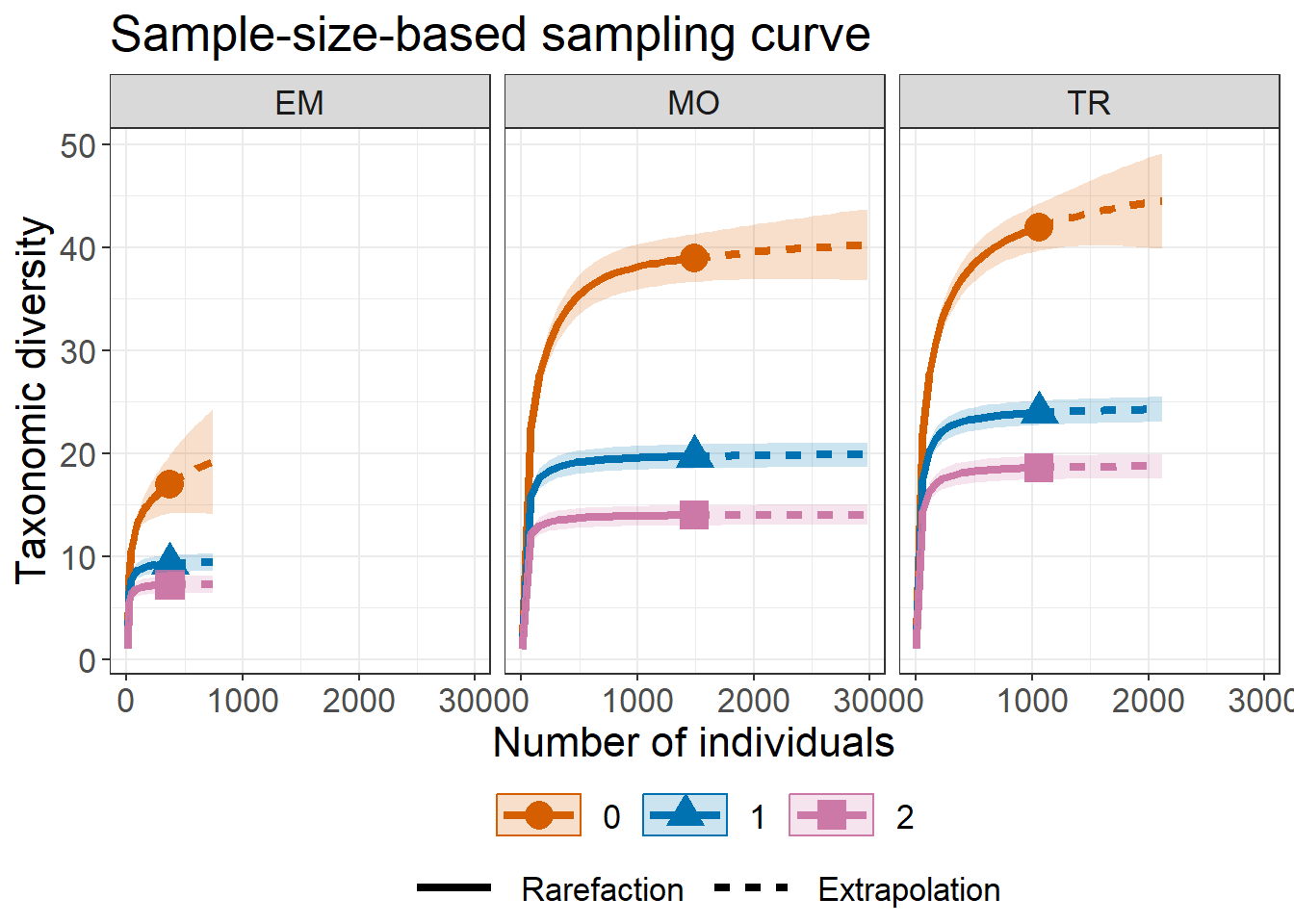When facet.var = "Order.q" in ggiNEXT3D function, it creates a separate plot for each diversity order and the different color lines represent each assemblage. Sample-size-based R/E curve (type = 1) as below:

# Sample-size-based R/E curves, separating by "Order.q"
ggiNEXT3D(out.TD, type = 1, facet.var = "Order.q")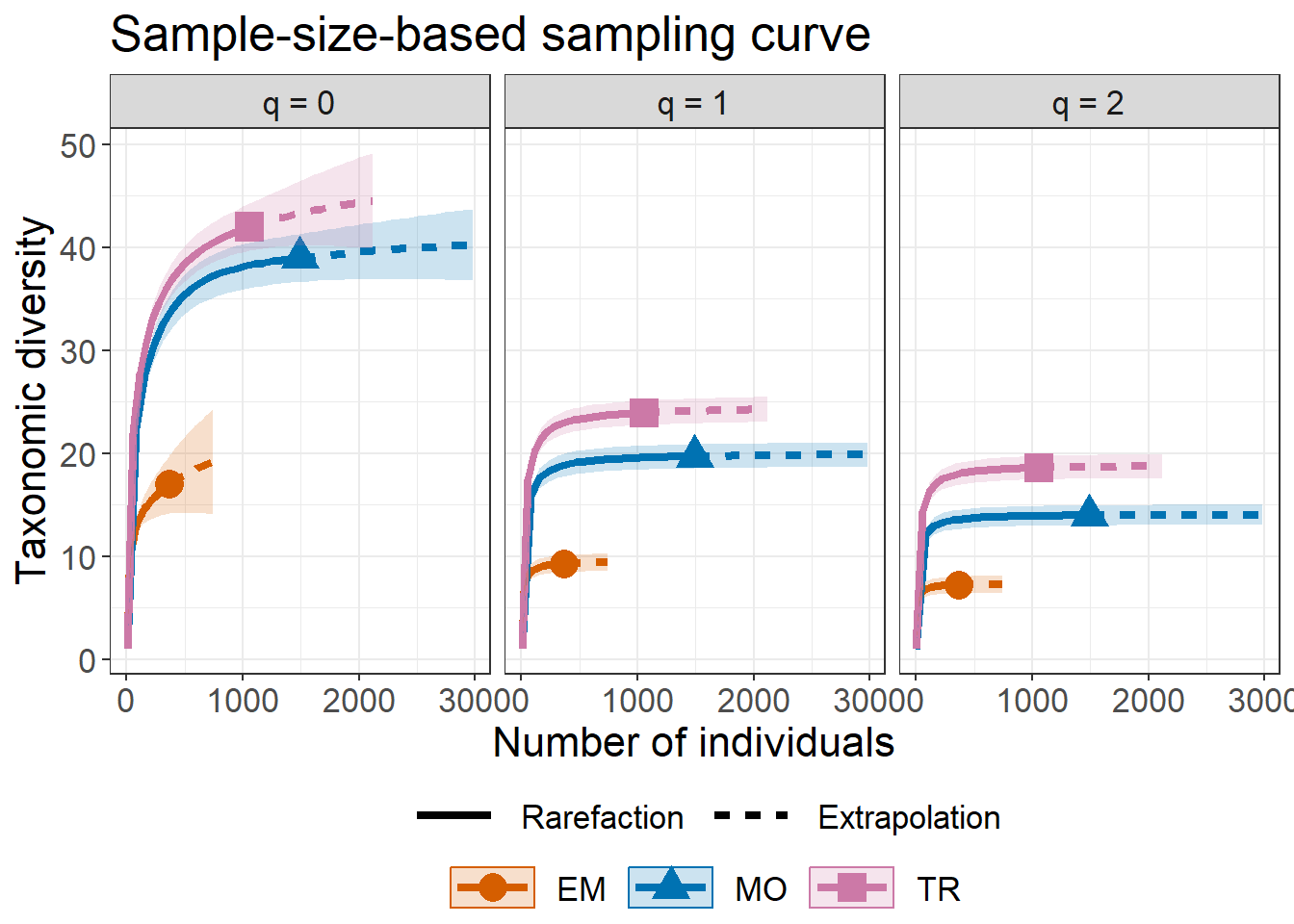The following command return the sample completeness (sample coverage) curve (type = 2) in which different colors are used for the three assemblages.

ggiNEXT3D(out.TD, type = 2, facet.var = "Order.q", color.var = "Assemblage")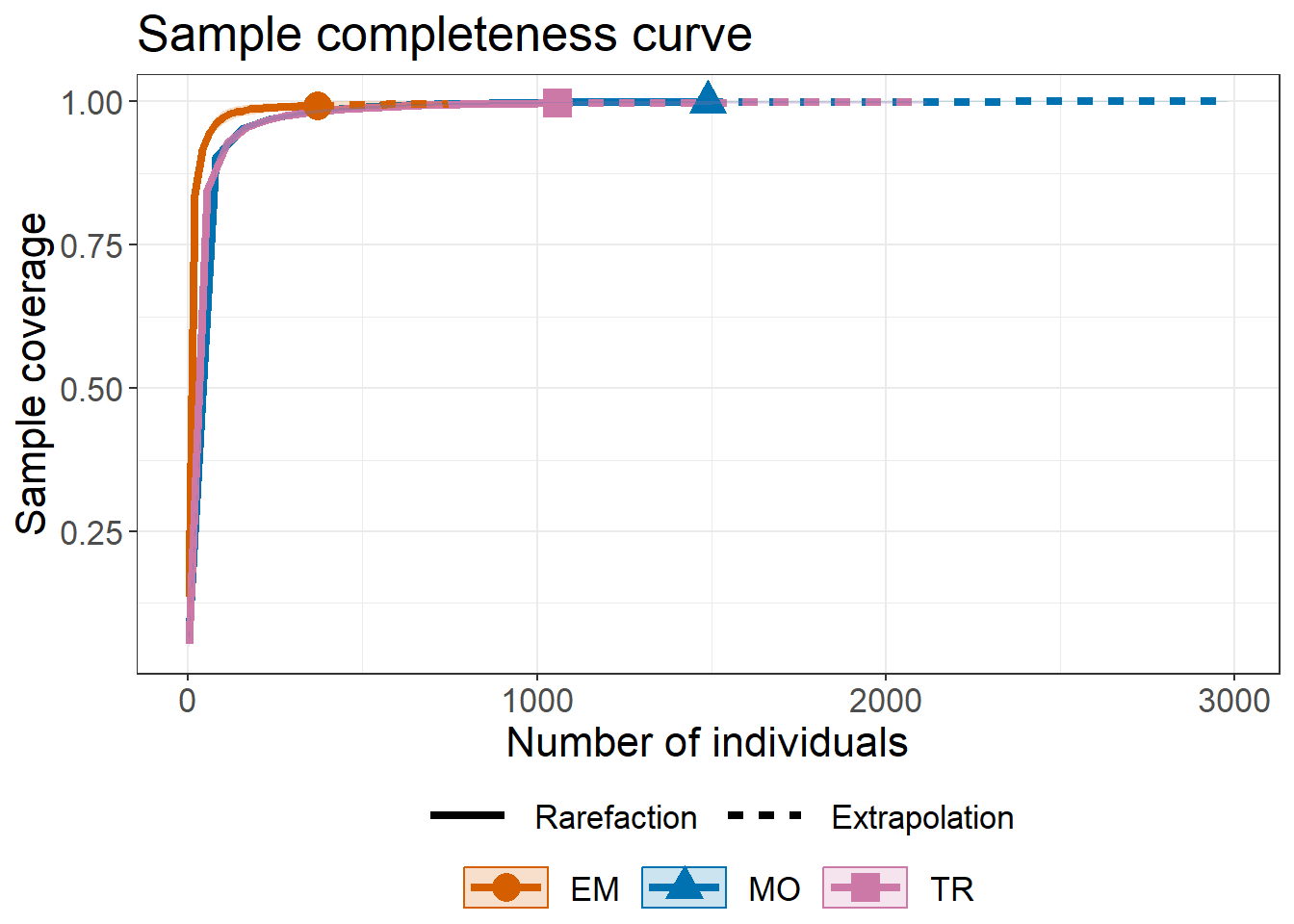The following commands return the coverage-based R/E sampling curves in which different colors are used for the three assemblages (facet.var = "Assemblage") and for three diversity orders (facet.var = "Order.q").

ggiNEXT3D(out.TD, type = 3, facet.var = "Assemblage")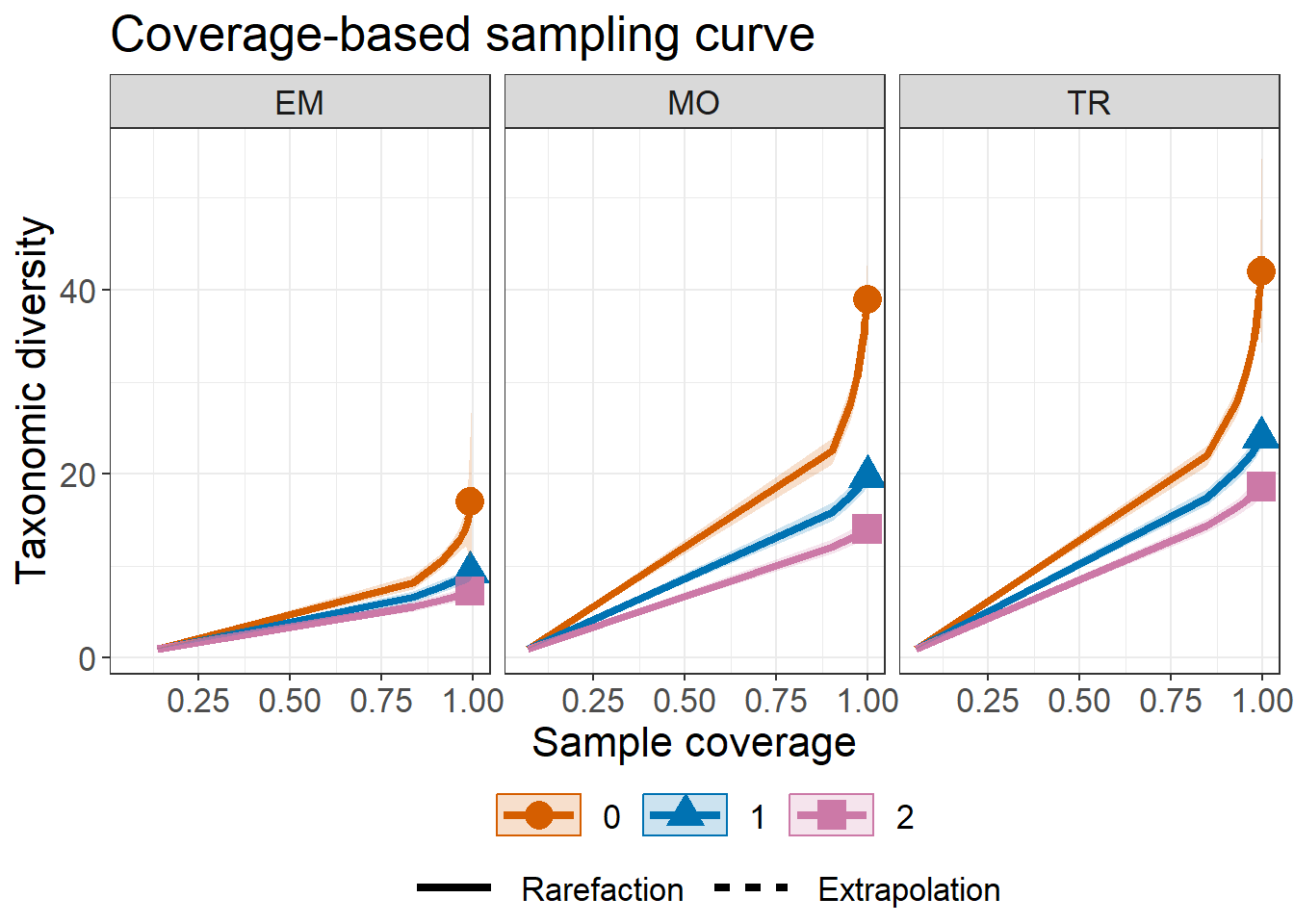ggiNEXT3D(out.TD, type = 3, facet.var = "Order.q")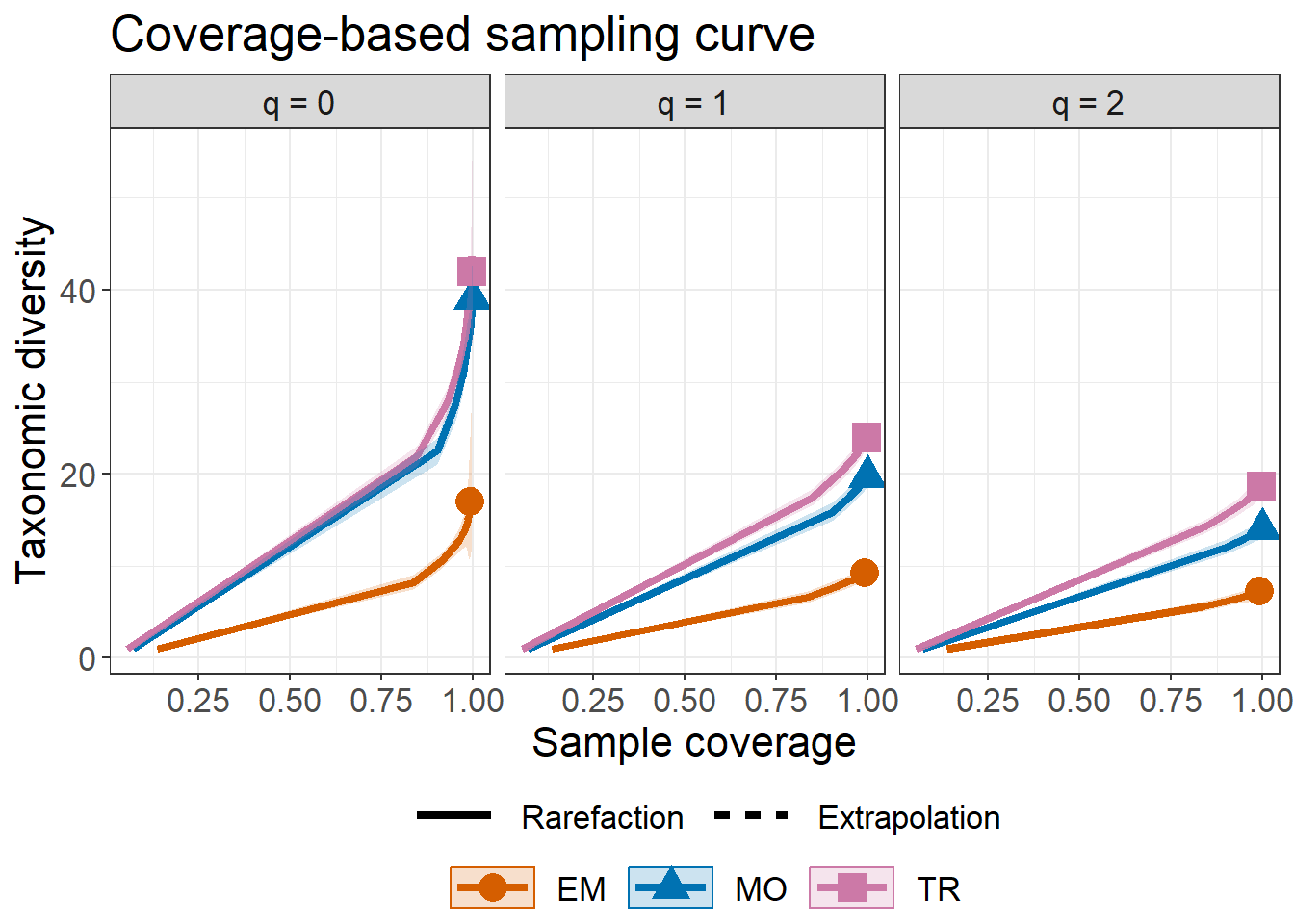### EXAMPLE for INCIDENCE-RAW DATA

Incidence raw data is allowed for three diversity dimensions. For illustration, use the Hinkley’s fish data (the dataset is included in the package) at three time periods (1981-1985, 1987-1991 and 2015-2019). This data set (fish) includes a list with three matrices; each matrix is a species-by-sampling-unit data. Here only use taxonomic diversity (TD) as demonstration below.

data("fish")
head(fish$data$1981-1985)
1 2 3 4 5 6 7 8 9 10 11 12 13 14 15 17 18 19 20 21 22 23 24 25 26 27 28 29 30 31 32 33 34 35 36 37 38 39 40 41 42 43 44 45 46 47 48 49 50 51 52 53 54 55 56 57 58 59 60 61
Agonus_cataphractus 0 0 1 0 0 0 0 0 0  0  0  0  0  0  0  0  0  0  0  0  1  1  0  0  1  0  1  1  1  0  0  0  0  1  0  0  0  0  0  0  0  0  0  0  0  1  0  1  0  0  1  0  1  0  0  0  0  1  1  0
Alosa_fallax        0 0 0 0 0 0 0 0 1  0  0  0  1  0  0  0  0  0  1  1  0  1  0  1  1  0  1  0  0  1  1  1  1  1  1  0  1  0  0  1  1  1  0  1  1  1  1  1  0  0  1  1  1  1  1  0  0  0  0  1
Ammodytes_marinus   0 0 0 0 0 0 0 0 0  0  0  0  0  0  0  0  0  0  0  0  0  0  0  0  0  0  0  0  0  0  0  0  0  0  0  0  0  0  0  0  0  0  0  0  0  0  0  0  0  0  0  0  0  0  0  0  0  0  0  0
Ammodytes_tobianus  0 0 0 0 0 0 1 0 0  0  0  1  0  0  0  0  0  0  0  0  0  0  0  0  0  0  0  0  0  0  0  0  0  1  0  0  0  0  0  0  0  0  0  1  0  0  0  0  0  0  0  0  0  0  0  0  0  0  0  0
Anguilla_anguilla   1 1 1 1 0 0 0 1 0  1  0  1  0  1  1  0  0  0  1  0  0  1  0  1  1  1  1  0  0  0  1  0  1  1  0  0  1  1  0  1  0  0  0  0  1  1  1  0  1  1  1  1  1  1  0  0  0  0  0  0
Aphia_minuta        0 0 0 0 1 1 0 0 0  0  0  0  0  0  1  1  1  1  1  0  0  0  0  0  0  0  1  1  1  1  1  0  1  0  0  0  0  0  0  0  1  1  1  0  0  0  0  0  0  0  1  1  1  1  1  1  0  0  0  0
# fish.tree = fish$tree ## for PD # fish.dis = fish$dist   ## for FD

out.raw <- iNEXT3D(data = fish$data, diversity = "TD", q = c(0, 1, 2), datatype = "incidence_raw", nboot = 30) ggiNEXT3D(out.raw, type = 1)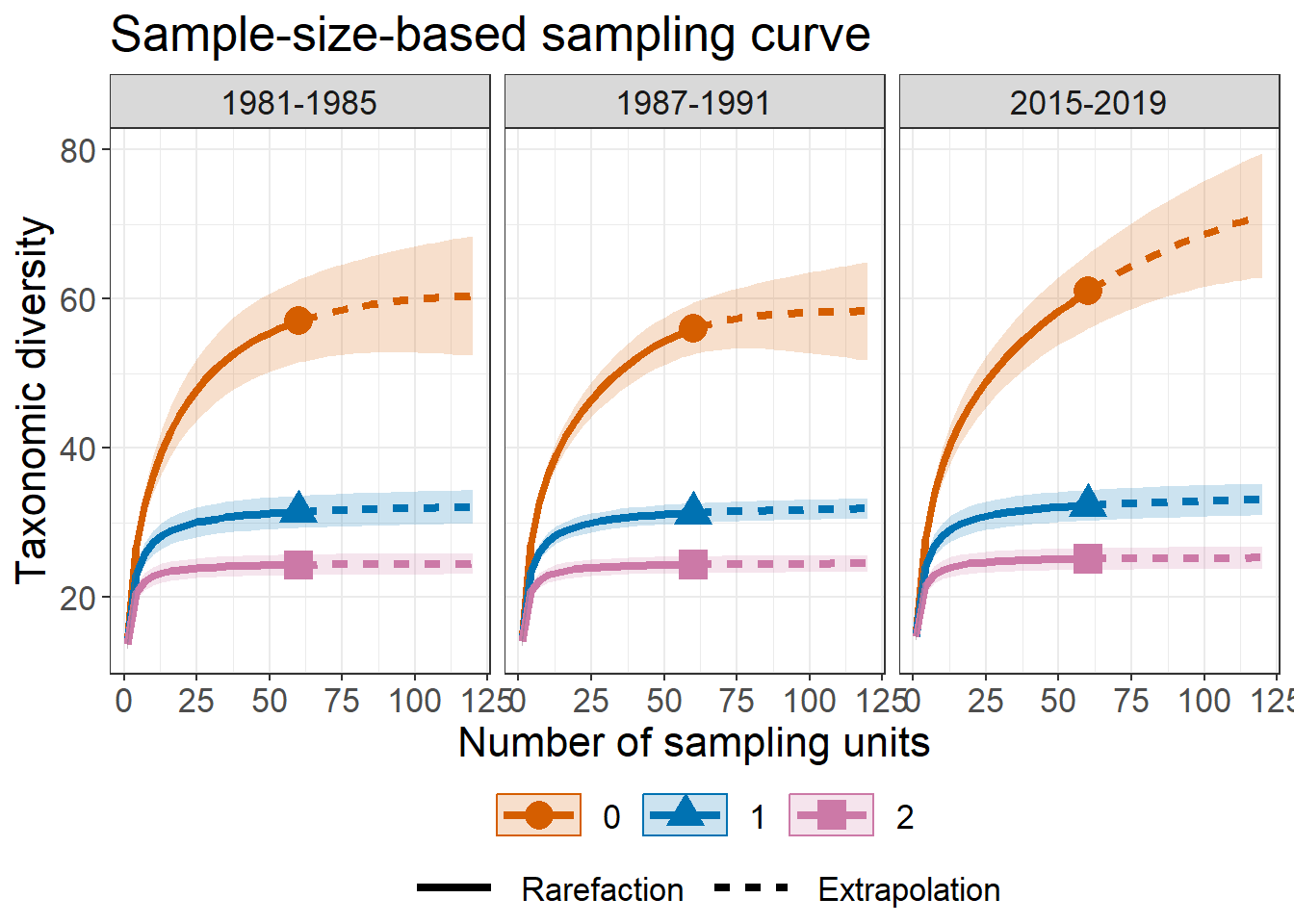ggiNEXT3D(out.raw, type = 2)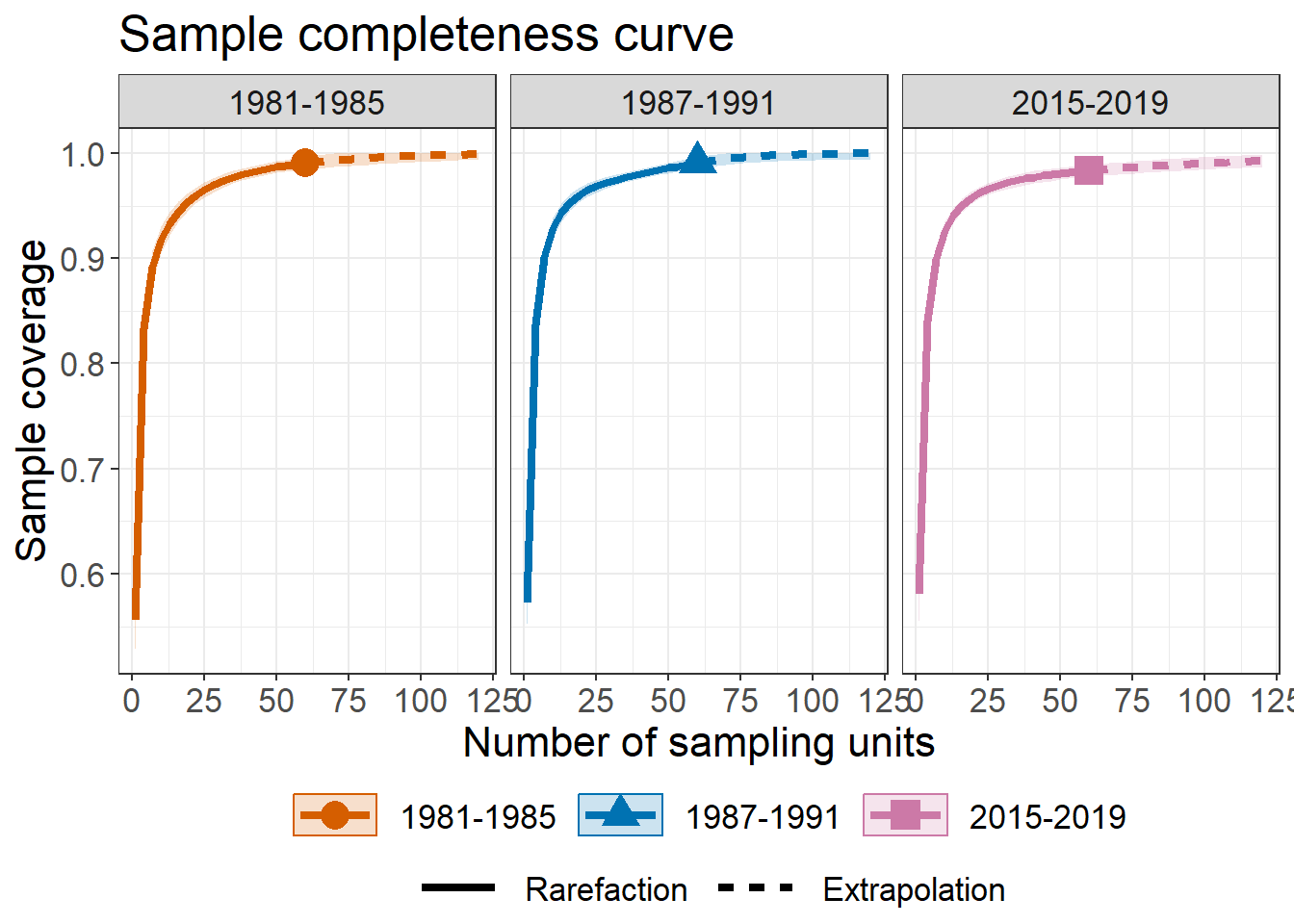ggiNEXT3D(out.raw, type = 3)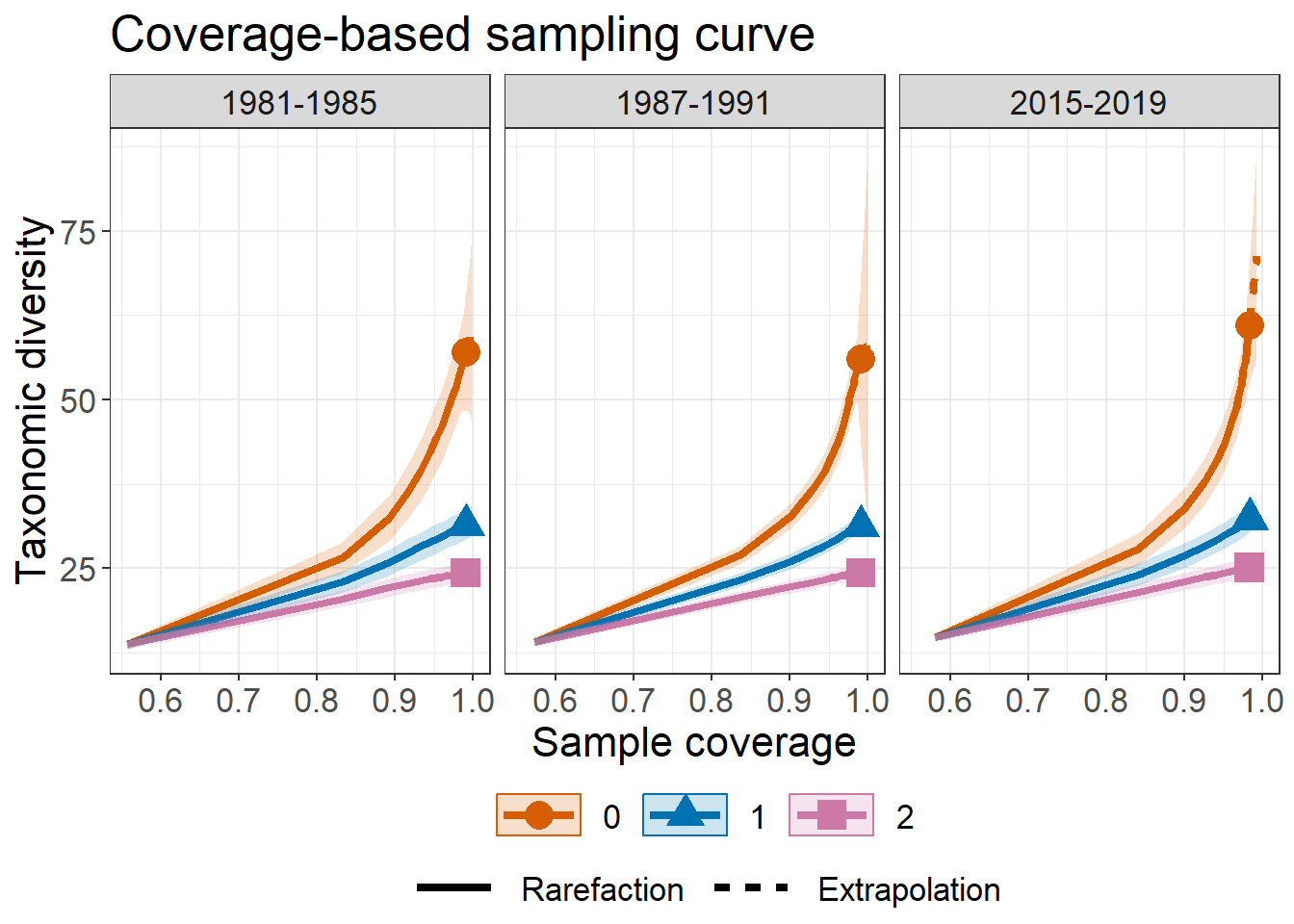### EXAMPLE for INCIDENCE-FREQUENCY DATA We transform Hinkley’s fish data (incidence-raw data) to incidence-frequency data (incidence_freq). The first row must be the total number of sampling units, followed by the species incidence frequencies in each assemblage. Here only use taxonomic diversity (TD) for demonstration below. fish.freq = cbind(c( ncol(fish$data$1981-1985), rowSums(fish$data$1981-1985) ), c( ncol(fish$data$1987-1991), rowSums(fish$data$1987-1991) ), c( ncol(fish$data$2015-2019), rowSums(fish$data$2015-2019) )) rownames(fish.freq) = "sample units" colnames(fish.freq) = names(fish$data)
head(fish.freq)
1981-1985 1987-1991 2015-2019
sample units               60        60        60
Agonus_cataphractus        14        19        21
Alosa_fallax               29        22        18
Ammodytes_marinus           0         0         0
Ammodytes_tobianus          4         2         8
Anguilla_anguilla          30        29         8

Note that incidence-frequency data (datatype = "incidence_freq) is allowed only for diversity class: "TD", "FD". If "PD" required, use incidence-raw data instead. The following commands return three R/E sampling curves for fish data. The argument color.var = "Order.q" is used to display curves in different colors for diversity order.

out.incfreq <- iNEXT3D(data = fish.freq, diversity = "TD",
q = c(0, 1, 2), datatype = "incidence_freq",nboot = 30)

# Sample-size-based R/E curves
ggiNEXT3D(out.incfreq, type = 1, color.var = "Order.q")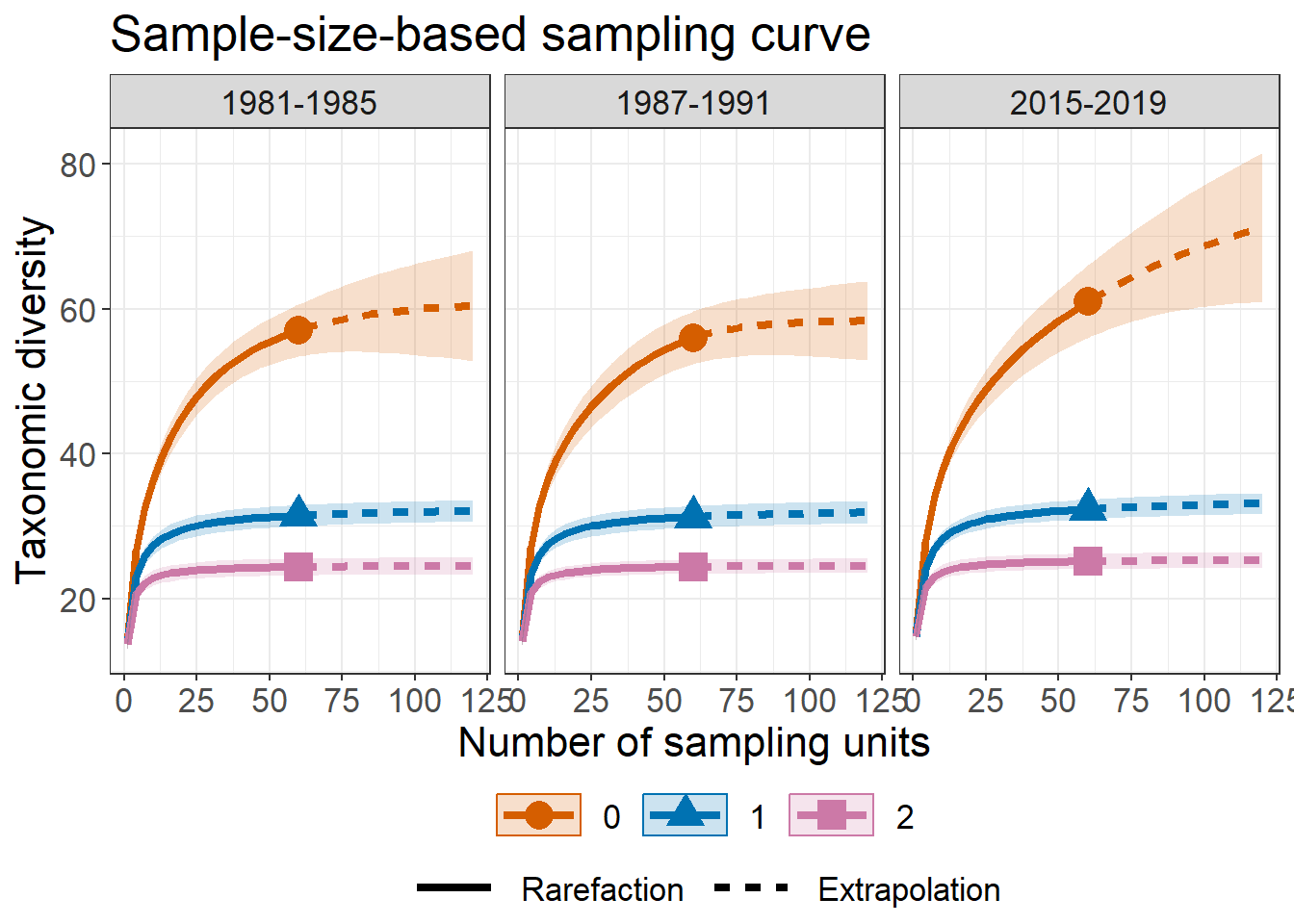# Sample completeness curves
ggiNEXT3D(out.incfreq, type = 2)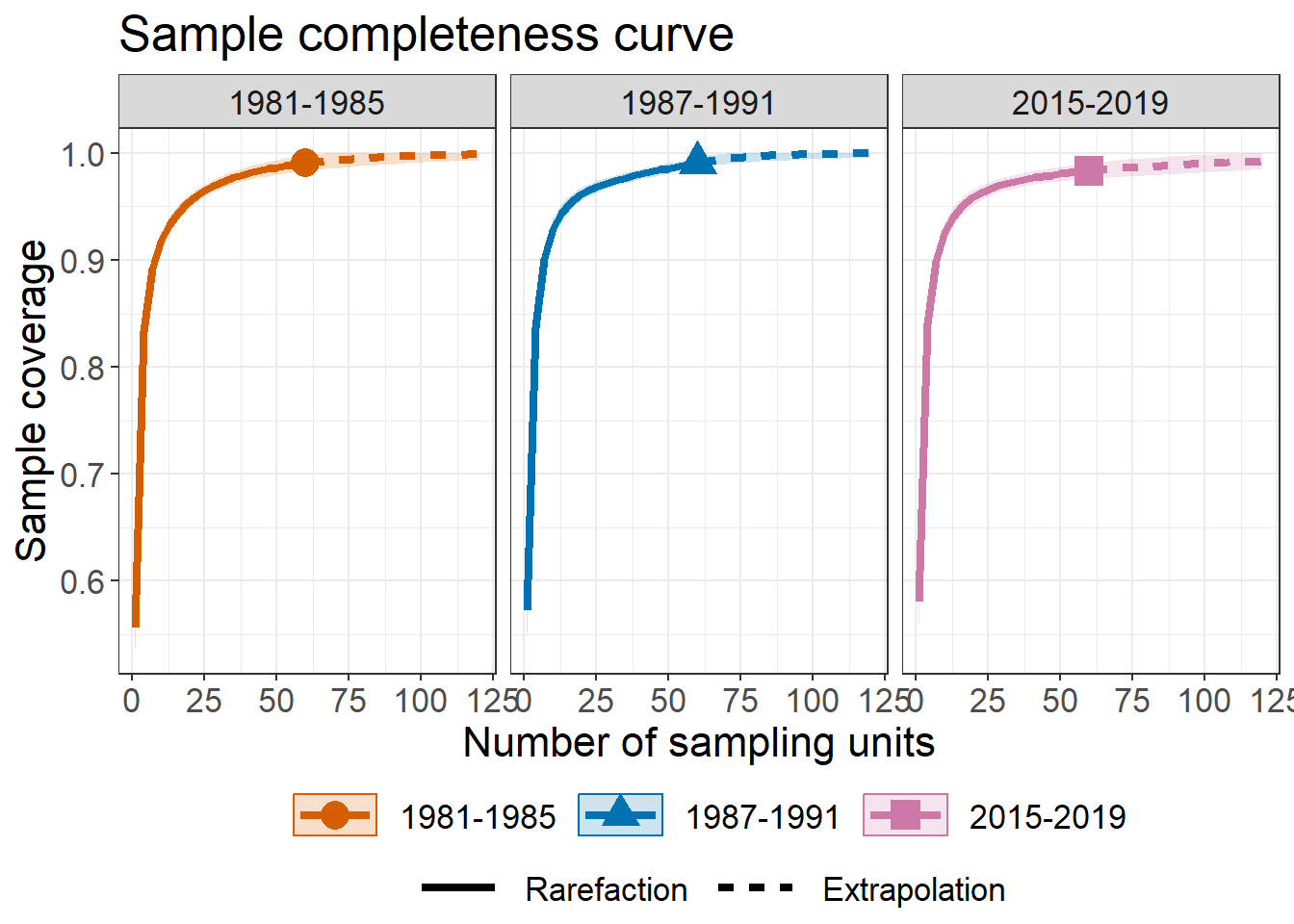# Coverage-based R/E curves
ggiNEXT3D(out.incfreq, type = 3, color.var = "Order.q")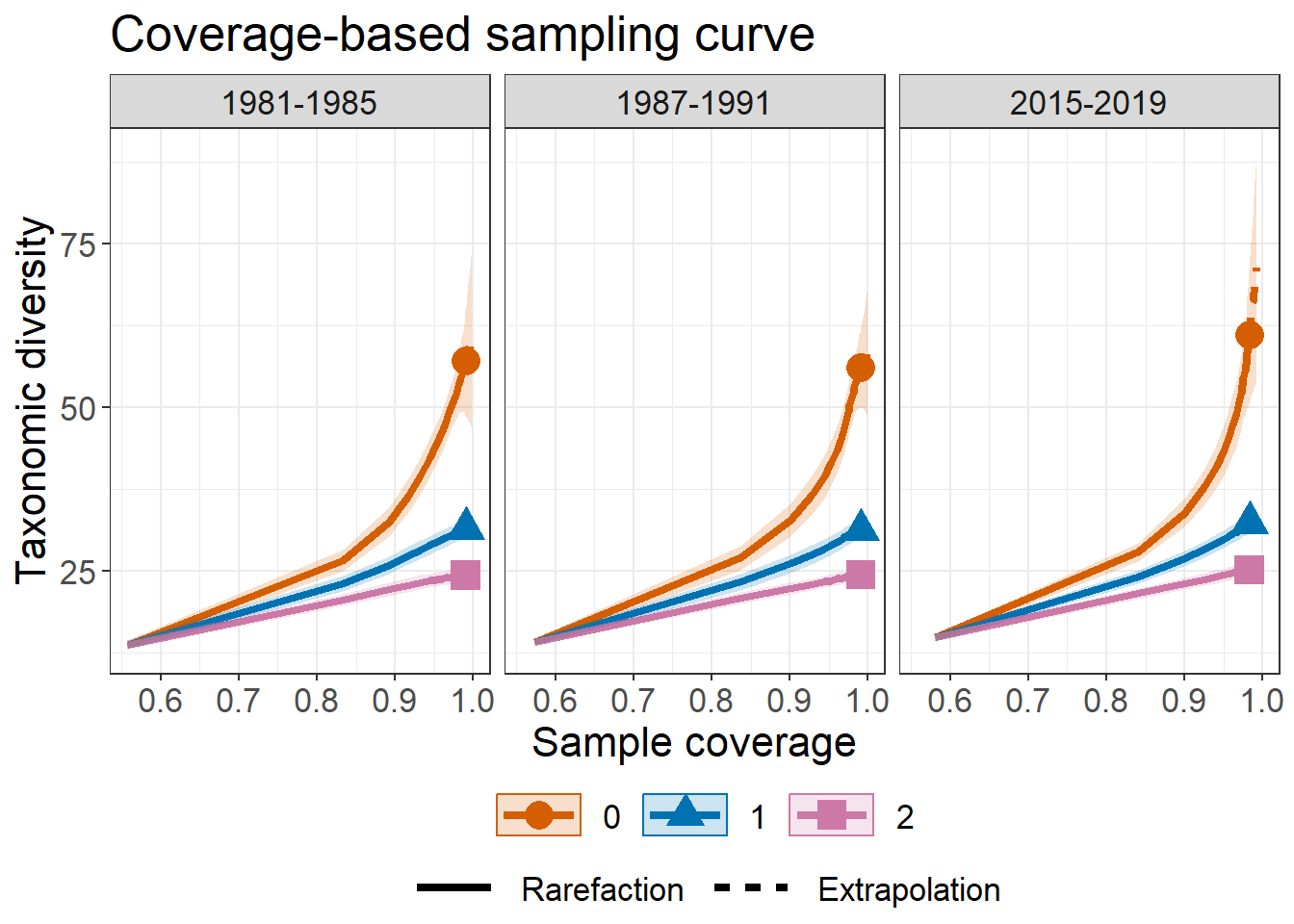## DATA INFORMATION FUNCTION: DataInfo3D()

DataInfo3D(data, diversity = "TD", datatype = "abundance",
nT = NULL, PDtree, PDreftime = NULL,
FDdistM, FDtype = "AUC", FDtau = NULL) 

Here provide the function DataInfo3D to compute three diversity dimensions (‘TD’, ‘PD’, ‘FD’) data information, which including sample size, observed species richness, sample coverage estimate, and the first ten abundance/incidence frequency counts when diversity = TD. And so on for PD, FD.

DataInfo3D(dunes$data, diversity = 'TD', datatype = "abundance") Assemblage n S.obs SC f1 f2 f3 f4 f5 f6 f7 f8 f9 f10 1 EM 373 17 0.9919715 3 1 1 0 0 1 0 1 1 1 2 MO 1490 39 0.9986586 2 1 1 4 2 2 3 1 1 1 3 TR 1059 42 0.9962264 4 2 4 2 2 2 0 1 2 2 ## POINT ESTIMATION FUNCTION: estimate3D() estimate3D(data, diversity = "TD", q = c(0, 1, 2), datatype = "abundance", base = "coverage", level = NULL, nboot = 50, conf = 0.95, nT = NULL, PDtree, PDreftime = NULL, PDtype = "meanPD", FDdistM, FDtype = "AUC", FDtau = NULL)  estimate3D is used to compute three diversity dimensions (TD, PD, FD) estimates with q = 0, 1, 2 under any specified level of sample size (when base = "size") or sample coverage (when base = "coverage") for either abundance data (datatype = "abundance") or incidence data (datatype = "incidence_freq" or "incidence_raw"). If level = NULL, this function computes the diversity estimates for the minimum sample size among all samples extrapolated to double reference sizes (when base = "size") or the minimum sample coverage among all samples extrapolated to double reference sizes (when base = "coverage"). For example, the following command returns the taxonomic diversity (‘TD’) with a specified level of sample coverage = 99.5% for the dunes data. For some assemblages, this coverage value corresponds to the rarefaction part whereas the others correspond to extrapolation. estimate3D(dunes$data, diversity = 'TD', q = c(0,1,2), datatype = "abundance",
base = "coverage", level = 0.995)
Assemblage        m        Method Order.q    SC        qD      s.e.    qD.LCL    qD.UCL
1         EM 637.4836 Extrapolation       0 0.995 18.692936 3.7151524 11.411371 25.974501
2         EM 637.4836 Extrapolation       1 0.995  9.400018 0.4263251  8.564436 10.235600
3         EM 637.4836 Extrapolation       2 0.995  7.268513 0.3410917  6.599986  7.937041
4         MO 732.4154   Rarefaction       0 0.995 37.191467 1.3095147 34.624866 39.758069
5         MO 732.4154   Rarefaction       1 0.995 19.428728 0.4145147 18.616294 20.241162
6         MO 732.4154   Rarefaction       2 0.995 13.855022 0.4035675 13.064045 14.646000
7         TR 853.3564   Rarefaction       0 0.995 41.115774 2.6535592 35.914893 46.316654
8         TR 853.3564   Rarefaction       1 0.995 23.815218 0.5957004 22.647667 24.982769
9         TR 853.3564   Rarefaction       2 0.995 18.531144 0.5996717 17.355809 19.706479

## EMPIRICAL AND ASYMPTOTIC DIVERSITY FUNCTION: AO3D

AO3D(
data, diversity = "TD", q = seq(0, 2, 0.2), datatype = "abundance",
nboot = 50, conf = 0.95, nT = NULL, method = c("Asymptotic", "Observed"),
PDtree, PDreftime = NULL, PDtype = "meanPD",
FDdistM, FDtype = "AUC", FDtau = NULL
)

The function AO3D() compute three diversity dimensions (TD, PD, FD) for empirical (observed) diversity and estimated asymptotic diversity with any diversity order. For example, the following commands returns empirical and asymptotic taxonomic diversity (‘TD’) for dunes data, along with its confidence interval at diversity order q from 0 to 2. Here only show the first ten rows.

out1 <- AO3D(dunes$data, diversity = 'TD', datatype = "abundance", method = c("Asymptotic", "Observed"), nboot = 30, conf = 0.95) head(out1, 10) Order.q qD s.e. qD.LCL qD.UCL Assemblage Method 1 0.0 21.487936 3.9145582 13.815543 29.160329 EM Asymptotic 2 0.2 16.936433 2.0626563 12.893701 20.979165 EM Asymptotic 3 0.4 13.885407 1.0562767 11.815142 15.955671 EM Asymptotic 4 0.6 11.862781 0.5891924 10.707985 13.017577 EM Asymptotic 5 0.8 10.496859 0.4118876 9.689575 11.304144 EM Asymptotic 6 1.0 9.541765 0.3605125 8.835173 10.248357 EM Asymptotic 7 1.2 8.847719 0.3517639 8.158274 9.537164 EM Asymptotic 8 1.4 8.325449 0.3555980 7.628489 9.022408 EM Asymptotic 9 1.6 7.920890 0.3628292 7.209758 8.632022 EM Asymptotic 10 1.8 7.600095 0.3706320 6.873669 8.326520 EM Asymptotic ## GRAPHIC DISPLAYS FUNCTION: ggAO3D() ggAO3D(outcome, profile = "q") ggAO3D plots q-profile, time-profile, and tau-profile based on ggplot2. Here outcome is the object from the function AO3D, and profile is a profile selection versus to diversity. Default is profile = "q". Note that profile = "time" is allowed for only when diversity = “PD” and profile = "tau" profile is allowed for only when diversity = "FD" and FDtype = "tau_values". # q-profile curves ggAO3D(out1,profile = "q")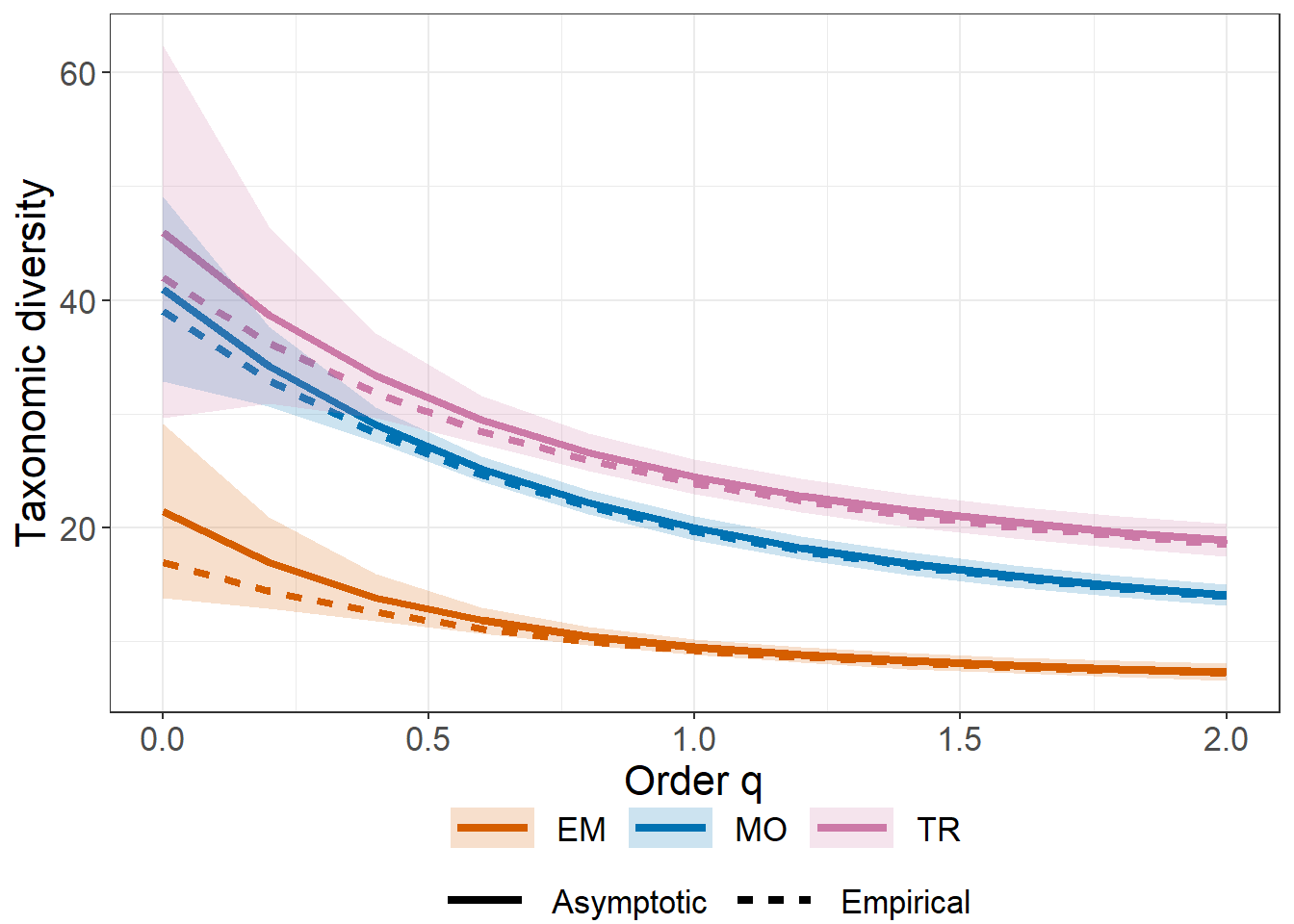The argument profile = "time" in ggAO3D function creates a separate plot for each diversity order q. Therefore the different assemblages will be represented by different color lines. # time-profile curves, separating by "Order.q" data(data.inc) data <- data.inc$data
tree <- data.inc$tree nT <- data.inc$nT

out2 <- AO3D(data, diversity = 'PD', q = c(0, 1, 2), datatype = "incidence_raw",
nT = nT, nboot = 30, method = c("Asymptotic", "Observed"),
PDtree = tree, PDreftime = seq(0.1, 82.8575, length.out = 40))
ggAO3D(out2, profile = "time")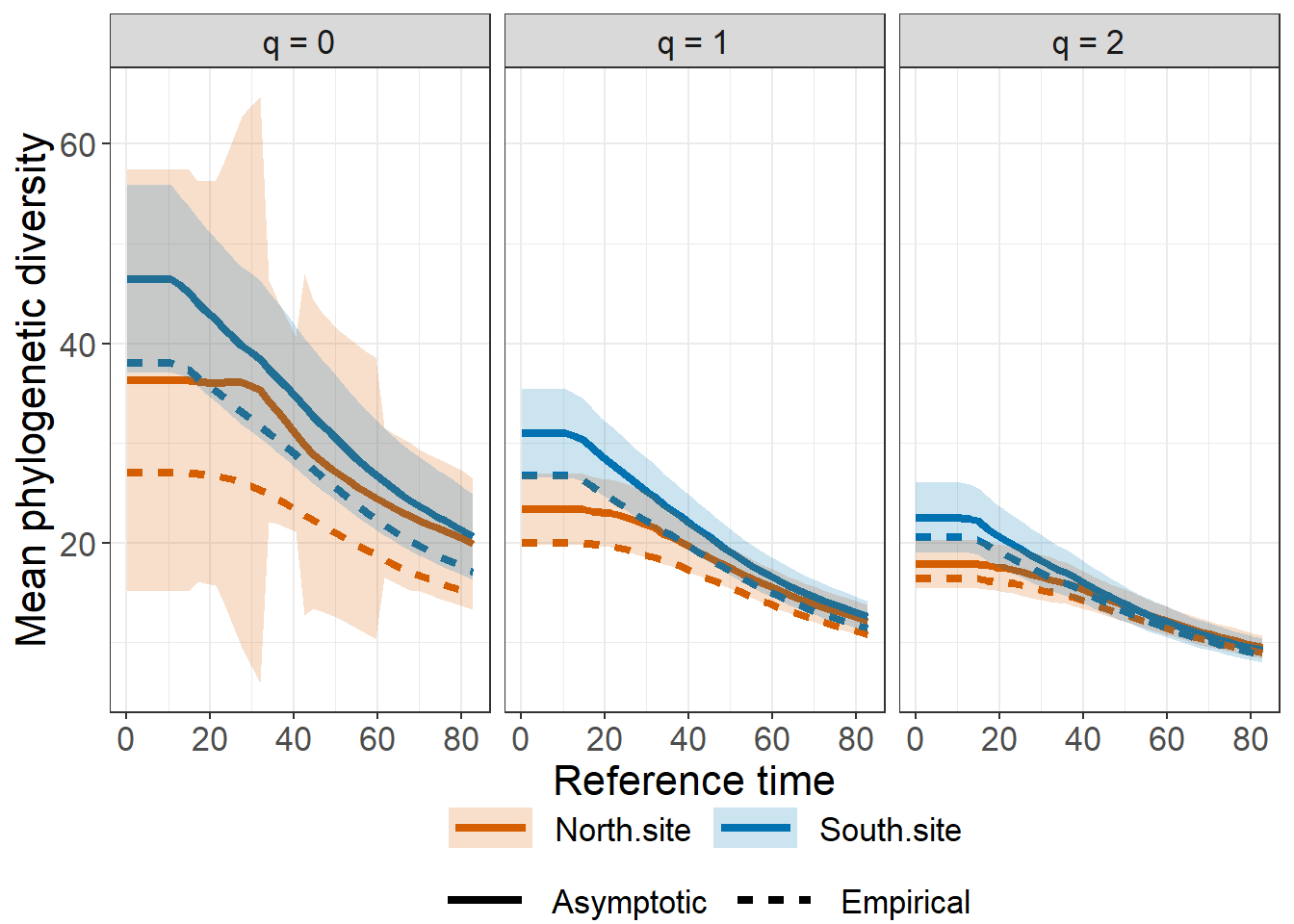The argument profile = "tau" in ggAO3D function creates a separate plot for each diversity order q. Therefore the different assemblages will be represented by different color lines.

# tau-profile curves, separating by "Order.q"
data <- dunes$data distM <- dunes$dist

out3 <- AO3D(data, diversity = 'FD', q = c(0, 1, 2), datatype = "abundance", nboot = 30, method = c("Asymptotic", "Observed"),
FDtau = seq(0, 1, 0.1), FDdistM = distM, FDtype = 'tau_values')
ggAO3D(out3, profile = "tau")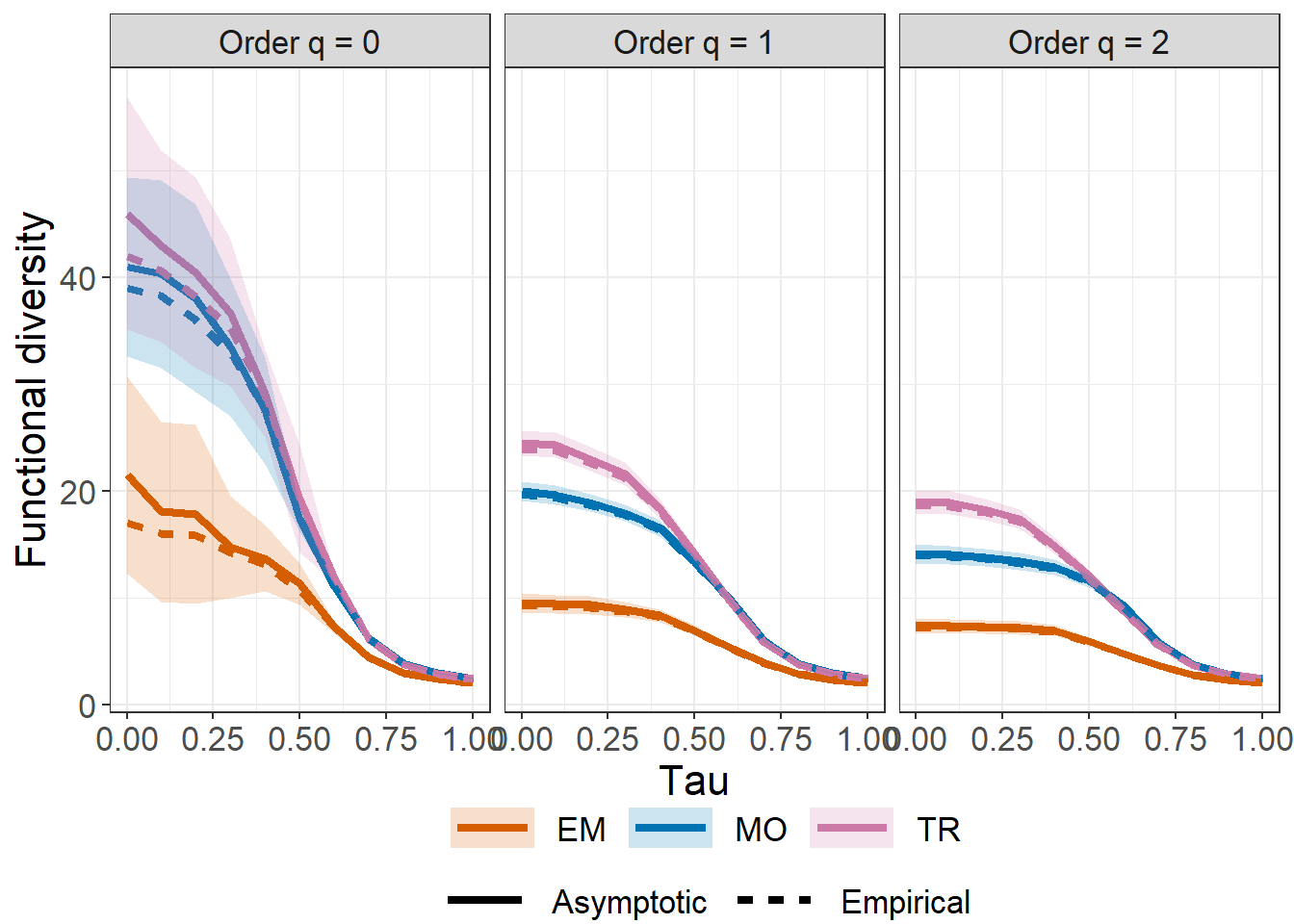The iNEXT.3D package is licensed under the GPLv3. To help refine iNEXT.3D, your comments or feedback would be welcome (please send them to Anne Chao or report an issue on the iNEXT.3D github iNEXT.3D_github.